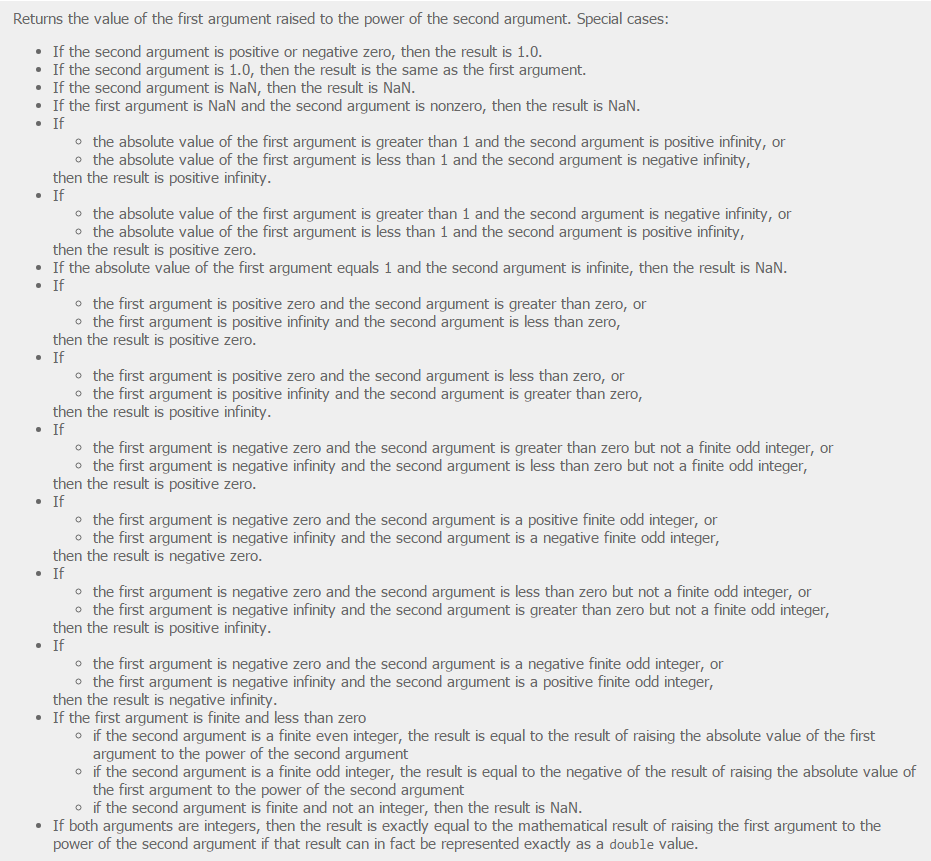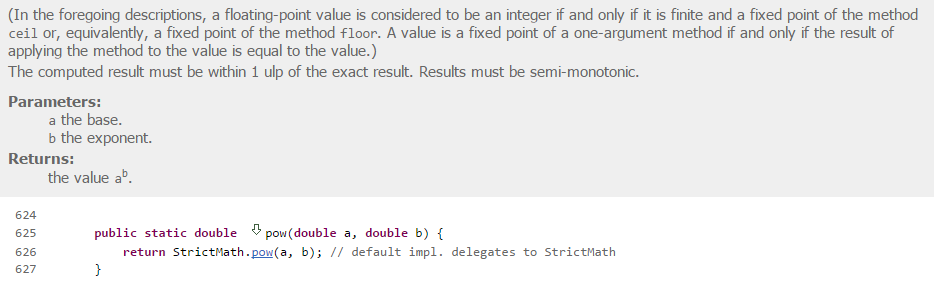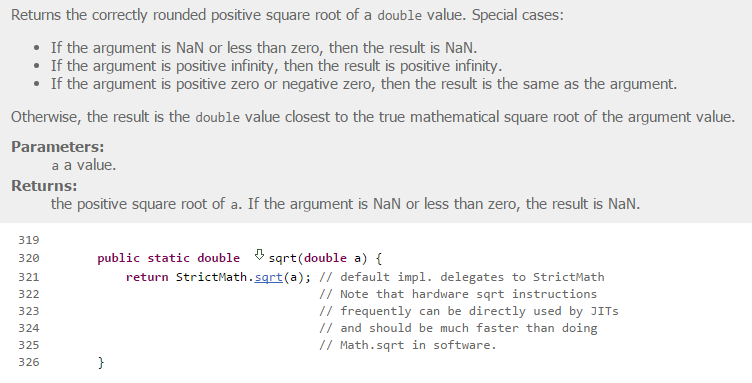# Games[Performance] Use (inlined) DistanceSquared to compare distances

#### wonder

##### Expert
Longtime User
Variables:
B4X:
``````'pX: Point X-coordinate
'pY: Point Y-coordinate
'cX: Circle center X-coordinate
'cY: Circle center Y-coordinate

B4X:
``````'- Function calls are expensive. We have 2: Power() and Sqrt()
'- The Power() function itself is more expensive than simple multiplication (see why below)
'- The Sqrt() function itself is an iterative function that should always be avoided.
Sub isPointInCircle(pX As Double, pY As Double, cX As Double, cY As Double, cR As Double) As Boolean
Return Sqrt(Power(cX - pX, 2) + Power(cY - pY, 2)) <= cR
End``````

Good:
B4X:
``````'- No function calls
'- No Sqrt()
'- No Power()
Sub isPointInCircle(pX As Double, pY As Double, cX As Double, cY As Double, cR As Double) As Boolean
'----------------------------------------
'DistanceSquared = (dX * dX) + (dY * dY)
'----------------------------------------
Dim dX = cX - pX As Double
Dim dY = cY - pY As Double
Return (dX * dX) + (dY * dY) <= (cR * cR)
End

'Note that I commented DistanceSquare and RadiusSquared,
'instead of declaring them as variables or function calls.``````

Now, let's have a look at the junk we just got rid of:

Power() function algorithm in Java:Sqrt() function algorithm in Java:Happy coding!Last edited:
•Danamo

#### sorex

##### Expert
Longtime User
what's the use of <= in this line?

B4X:
``Return Sqrt(Power(cX - pX, 2) + Power(cY - pY, 2)) <= cR``

#### wonder

##### Expert
Longtime User
@sorex, it allows the (Boolean) function to return whether or nor the distance is less or equal than the radius (as either True or False).
Using an IF statement to return a boolean which stems from a binary decision ("either this or that"), is in my opinion, very amateurish.Ugly:
B4X:
``````If a > b Then
Return True
Else
Return False
End If``````

Pretty:
B4X:
``Return a > b``

Last edited:

#### sorex

##### Expert
Using an IF statement to return a boolean which stems from a binary decision ("either this or that"), is very amateurish.I know someone who would answer "it reads easier"•